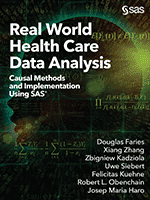Do you wish you could predict the likelihood that one of your customers will open your marketing email? Or what if you could tell whether a new medical treatment for a patient will have a better outcome than the standard treatment? If you are familiar with propensity modeling, then you know such predictions about future behavior are possible! Propensity models generate a propensity score, which is the probability that a future behavior will occur. Propensity models are used often in machine learning and predictive data analytics, particularly in the fields of marketing, economics, business, and healthcare. These models can detect and remove bias in analysis of real-world, observational data where there is no control group.

SAS provides several approaches for calculating propensity scores. This excerpt from the new book, Real World Health Care Data Analysis: Causal Methods and Implementation Using SAS®, discusses one approach for estimating propensity scores and provides associated SAS code. The example code and data used in the examples is available to download here.

### A priori logistic regression model

One approach to estimating a propensity score is to fit a logistic regression model a priori, that is, identify the covariates in the model and fix the model before estimating the propensity score. The main advantage of an a priori model is that it allows researchers to incorporate knowledge external to the data into the model building. For example, if there is evidence that a covariate is correlated to the treatment assignment, then this covariate should be included in the model even if the association between this covariate and the treatment is not strong in the current data. In addition, the a priori model is easy to interpret. The directed acyclic graph approach could be very informative in building a logistic propensity score model a priori, as it clearly points out the relationship between covariates and interventions. The correlation structure between each covariate and the intervention selection is pre-specified and in a fixed form. However, one main challenge of the a priori modeling approach is that it might not provide the optimal balance between treatment and control groups.

### Building an a priori model

To build an a priori model for propensity score estimation in SAS, we can use either PROC PSMATCH or PROC LOGISTIC as shown in Program 1. In both cases, the input data set is a one observation per patient data set containing the treatment and baseline covariates from the simulated REFLECTIONS study. Also, in both cases the code will produce an output data set containing the original data set with the additional estimated propensity score for each patient (_ps_).

Program 1: Propensity score estimation: a priori logistic regression

```PROC PSMATCH DATA=REFL2 REGION=ALLOBS;
CLASS COHORT GENDER RACE DR_RHEUM DR_PRIMCARE;
PSMODEL COHORT(TREATED='OPIOID')= GENDER RACE AGE BMI_B BPIINTERF_B BPIPAIN_B
CPFQ_B FIQ_B GAD7_B ISIX_B PHQ8_B PHYSICALSYMP_B SDS_B DR_RHEUM
DR_PRIMCARE;
OUTPUT OUT=PS PS=_PS_;
RUN;

PROC LOGISTIC DATA=REFL2;
CLASS COHORT GENDER RACE DR_RHEUM DR_PRIMCARE;
MODEL COHORT = GENDER RACE AGE BMI_B BPIINTERF_B BPIPAIN_B CPFQ_B FIQ_B GAD7_B
ISIX_B PHQ8_B PHYSICALSYMP_B SDS_B DR_RHEUM DR_PRIMCARE;
OUTPUT OUT=PS PREDICTED=PS;
RUN;```

Before building a logistic model in SAS, we suggest examining the distribution of the intervention indicator at each level of the categorical variable to rule out the possibility of “complete separation” (or “perfect prediction”), which means that for subjects at some level of some categorical variable, they would all receive one intervention but not the other. Complete separation can occur for several reasons and one common example is when using several categorical variables whose categories are coded by indicators. When the logistic regression model is fit, the estimate of the regression coefficients βs is based on the maximum likelihood estimation, and MLEs under logistic regression modeling do not have a closed form. In other words, the MLE β̂ cannot be written as a function of Xi and Ti. Thus, the MLE of βs are obtained using some numerical analysis algorithms such as the Newton-Raphson method. However, if there is a covariate X that can completely separate the interventions, then the procedure will not converge in SAS. If PROC LOGISTIC was used, the following warning message will be issued.

```WARNING: There is a complete separation of data points. The maximum likelihood estimate does not exist.

WARNING: The LOGISTIC procedure continues in spite of the above warning. Results shown are based on the last maximum likelihood iteration. Validity of the model fit is questionable.```

Notice that SAS will continue to finish the computation despite issuing warning messages. However, the estimate of such βs are incorrect, and so are the estimated propensity scores. If after examining the intervention distribution at each level of the categorical variables complete separation is found, then efforts should be made to address this issue. One possible solution is to collapse the categorical variable causing the problem. That is, combine the different outcome categories such that the complete separation no longer exists.

### Firth logistic regression

Another possible solution is to use Firth logistic regression. It uses a penalized likelihood estimation method. Firth bias-correction is considered an ideal solution to the separation issue for logistic regression (Heinze and Schemper, 2002). In PROC LOGISTIC, we can add an option to run the Firth logistic regression as shown in Program 2.

Program 2: Firth logistic regression

```PROC LOGISTIC DATA=REFL2;
CLASS COHORT GENDER RACE DR_RHEUM DR_PRIMCARE;
MODEL COHORT = GENDER RACE DR_RHEUM DR_PRIMCARE BPIInterf_B BPIPain_B
CPFQ_B FIQ_B GAD7_B ISIX_B PHQ8_B PhysicalSymp_B SDS_B / FIRTH;
OUTPUT OUT=PS PREDICTED=PS;
RUN;```

### References

Heinze G, Schemper M (2002). A solution to the problem of separation in logistic regression. Statistics in Medicine 21.16: 2409-2419.

Share